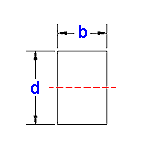# Section Properties: Rectangle at Center

 Variables inputs: Inch (in.) Metric (mm) "d" (Height) "b" (Width) Calculations Moment of Inertia (Units4) = Section Modulus (Units3) = Radius of Gyration (Units) = Extreme Point (Units) = Area (Units2) =